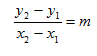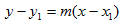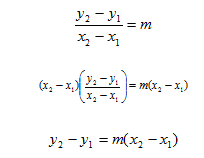Introduction

Remember that slope is rise/run. The change in y-values between two points on the line represents the rise and the change in the x -values between the same two points determines the run. Therefore the slope of a line given two points on the line can be found by using the following equation:When the coordinates of a point and the slope of a line are known, the line can be represented in point-slope form. The point-slope formula isand is derived from the equation of slope.This is a formula to find an equation of a line when given a point and the slope.

Listen